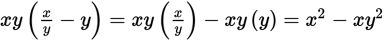# SAT Math Multiple Choice Question 883: Answer and Explanation

### Test Information

Question: 883

13.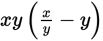Which of the following is equivalent to the expression above?

• A.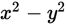• B.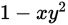• C.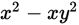• D.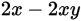Explanation:

C

Difficulty: Medium

Category: Passport to Advanced Math / Exponents

Strategic Advice: When a product contains parentheses, and none of the terms inside the parentheses are like terms, chances are that you need to apply the distributive property. Then simplify using the rules of exponents.

Getting to the Answer: Rather than distributing mentally, rewrite the expression so that xy is multiplied by each term inside the parentheses: